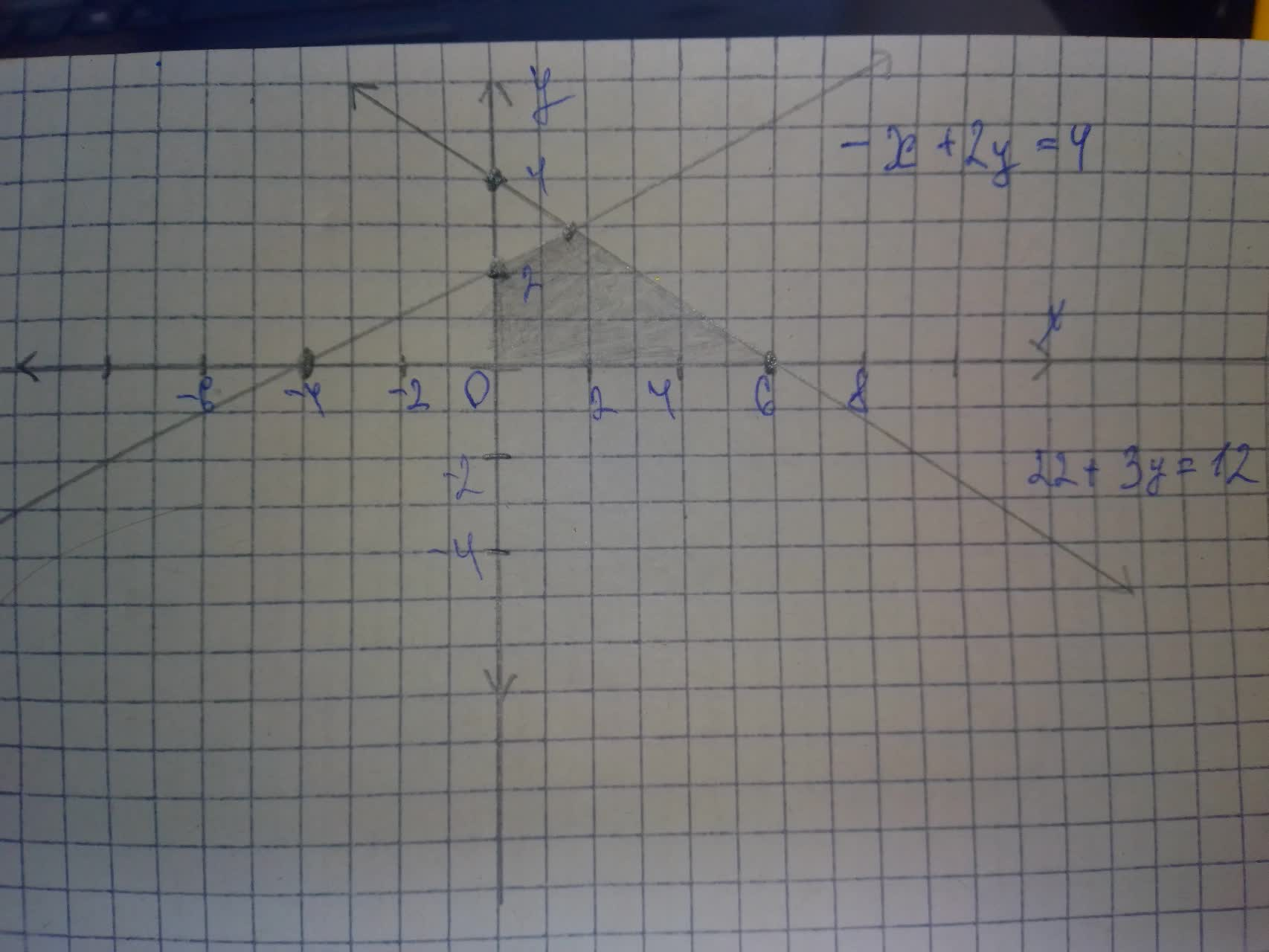e1s2kat26

2021-07-31

4.(a) Sketch the region of solutions of the following systems of inequalities:
$2?+3?\le 12$
$-?+2?<4$
$?\ge 0$
$?\ge 0$
b. List the coordinates of all of the corner points:
c Which corner point would maximize the equation $?=5?+3??$Laaibah Pitt

Step 1
Inequalities
Step 2
$2x+3y\le 12$
$-x+2y<4$
$x\ge 0$
$y\ge 0$
$\begin{array}{|ccc|}\hline x& 0& 6\\ 4& 4& 0\\ \hline\end{array}$
$\begin{array}{|ccc|}\hline x& 0& -4\\ 4& 2& 0\\ \hline\end{array}$Intersection pt: $2x+3y=12$
$-2x+4y=8$
$7y=20$
$y=\frac{20}{7}$
$x=\frac{12}{7}$
b) Comer points: $\left(\frac{12}{7},\frac{20}{7}\right),\left(6,0\right),\left(0,2\right),\left(0,0\right)$
c) $z=5x+3y$
$A\left(6,0\right),z=30$ (Max)
$B\left(0,2\right),z=6$
$C\left(0,0\right),z=0$
$D\left(\frac{12}{7},\frac{20}{7}\right)mz=\frac{120}{7}$

Do you have a similar question?